# Fibonacci numbers

(diff) ← Older revision | Latest revision (diff) | Newer revision → (diff)

The elements of the sequence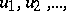given by the initial valuesand the recurrence relation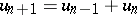. The first 14 Fibonacci numbers were produced for the first time in 1228 in the manuscripts of Leonardo da Pisa (Fibonacci).

Operations that can be performed on the indices of the Fibonacci numbers can be reduced to operations on the numbers themselves. The basis for this lies in the "addition formula" :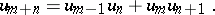Immediate corollaries of it are:etc. The general "multiplication formula" is more complicated: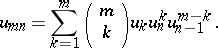The elementary divisibility properties of the Fibonacci numbers are mainly determined by the following facts:; ifis a prime number of the form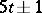, then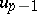is divisible by, while if it is of the form, thenis; if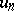is divisible by a prime numberand if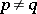, thenis not divisible by; ifis divisible by a prime number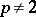, then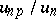is divisible by, but not by; ifis divisible by 4, then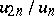is divisible by 2, but not by 4; ifis divisible by 2 but not by 4, thenis divisible by 4, but not by 8. At the same time, some number-theoretic problems connected with Fibonacci numbers are extremely hard. For example, the question of whether the set of prime Fibonacci numbers is finite or not has not been solved (1984).

An important role in the theory of Fibonacci numbers is played by the number, which is a root of the equation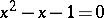. Thus Binet's formula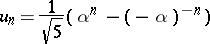holds; it implies thatis the nearest integer to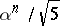, and thatThe Fibonacci numbers occupy a special position in the theory of continued fractions. In the continued-fraction expansion of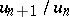all the partial quotients are ones and the number of them is not less than that of the incomplete quotients of the expansion of any other fraction with denominator less than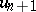. In a certain sense the numberis described by its approximating fractionsin a "worst possible" way.

How to Cite This Entry:
Fibonacci numbers. Encyclopedia of Mathematics. URL: http://encyclopediaofmath.org/index.php?title=Fibonacci_numbers&oldid=12076
This article was adapted from an original article by N.N. Vorob'ev (originator), which appeared in Encyclopedia of Mathematics - ISBN 1402006098. See original article